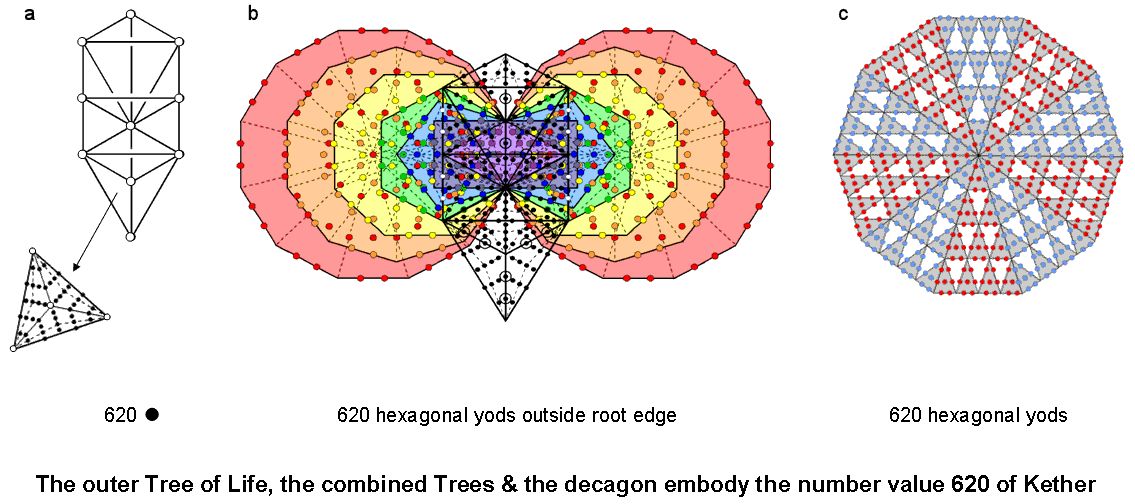<< Previous    1...   20  21    23  24  ...38    Next >>

#22 The outer Tree of Life with Type B triangles & the combined Trees of Life with Type A polygons embody the number 620 of Kethera) Outer Tree of Life
The Type B triangle has 46 yods (see here). Nine yods line its perimeter, which encloses 37 yods. Therefore, 36 internal yods surround its centre. There are 16 triangles in the outer Tree of Life. When they are Type B, they have (36×16 = 576 = 242 = 12×22×32×42) internal yods other than their (42 = 16) centres, as well as (22×2 = 44) hexagonal yods on their 22 sides other than their (1+2+3+4=10) corners. This illustrates how the Tetrad expresses the various yod populations of the outer Tree of Life with Type B triangles. The total number of yods other than the corners and centres of these 16 triangles = 576 + 44 = 620. This is the gematria number value of Kether (see here), the first Sephirah of the Tree of Life: KTR = 20 + 400 + 200 = 620 (see #5).

b) Combined Trees of Life
The Type A triangle has 19 yods (see here). Inside it are ten yods. Inside the 16 Type A triangles of the outer Tree of Life are (16×10 = 160) yods that comprise 16 corners of tetractyses and (160−16=144) hexagonal yods. 44 hexagonal yods are on their 22 sides. The number of hexagonal yods in the outer Tree of Life = 144 + 44 = 188. They comprise 140 hexagonal yods on the 70 sides of the 48 tetractyses and 48 hexagonal yods at the centres of the latter. 140 is the number value of Masloth, the Mundane Chakra of Chokmah, and 48 is the number of Kokab, the Mundane Chakra of Hod.

The inner Tree of Life has 444 hexagonal yods when the 94 sectors of its (7+7) enfolded polygons are turned into tetractyses (see here). When they are superposed onto the outer Tree, the centres of the two enfolded triangles, which are corners of tetractyses, coincide with the two hexagonal yods on the Path connecting Chesed and Geburah, thereby removing them from the count of the total number of hexagonal yods in the combined outer & inner Trees, although they remain, of course, hexagonal yods as far as the former is concerned. Four white hexagonal yods on each side pillar coincide with hexagonal yods on the vertical, internal sides of the sectors of the two hexagons. The total number of hexagonal yods in the combined outer & inner Trees of Life = 188 − 2 + 444 − 4 − 4 = 622. There are 620 hexagonal yods other than the two hexagonal yods on the root edge of the (7+7) enfolded polygons.

c) Decagon
Suppose that each sector of the decagon is turned into a 2nd-order tetractys. 720 yods surround its centre, of which (100 = 13 + 23 + 33 + 43) yods are corners of 10 tetractyses and 620 yods are hexagonal yods (see #5). The factor of 10 in this polygonal representation of the number of Kether arises from the 10 sides of the decagon, there being 72 yods and 62 hexagonal yods per sector, where 72 is the number of Chesed and 62 is the number value of Tzadkiel, the Archangel of this Sephirah. That this factor should aptly appear also in the 10-fold outer Tree of Life, as well as in its combination with its inner form, is non-trivial. These numbers illustrate the geometrical basis of the Kabbalistic system of Sephiroth, their Divine Names, etc and their gematria number values.

The outer Tree of Life has 214 yods. 16 yods (seven yods on each side pillar and the two hexagonal yods on the Path connecting Chesed and Geburah) are shared with the (7+7) enfolded polygons, which have 524 yods. The combined outer & inner Trees of Life contain (214−16+524=722) yods. Ten of these are Sephiroth and another 12 yods are centres of polygons. Therefore, there are (722−10−12=700) yods other than Sephiroth or centres. The significance of this is that 700 = 70×10, where 70 is the number of yods in the outer Tree of Life with its triangles turned into tetractyses. The combined Trees are composed of (48+94=142) tetractyses, each with a hexagonal yod at its centre. The number of yods lining their 241 sides = 722 − 142 = 580. Outside the root edge are 576 yods on 240 sides of 142 tetractyses, where 576 = 242 = 12×22×32×42. This illustrates once again how the Tetrad expresses properties of sacred geometries. The number of yods in the combined Trees that are unshared with each other = 722 − 16 = 706. There are 702 unshared yods outside the root edge, where

702 = 2×351 = 2(1 + 2 + 3 + ... + 26) = 2 + 4 + 6 + ... + 52

is the sum of the first 26 even integers, 351 is the number of Ashim, the Order of Angels assigned to Malkuth, and 26 is the number of YAHWEH. This shows how this Godname prescribes the number of unshared yods outside the root edge. As

700 = 702 − 2 = 4 + 6 + 8 + ... + 52,

we see that the sum of all the even integers, starting with the Tetrad, up to 52 (the 26th even integer) is the number of yods in the combined Trees of Life other than Sephiroth and centres of the 14 polygons of the inner Tree. In the discussion of the Tetrahedral Lambda (see Figure 5 here), it was pointed out that the sum of the 20 integers in the tetrahedral array is 350, whilst the sum of the integers in its four separate faces is 700. This sum is, therefore, the number of yods in the combined Trees other than Sephiroth and centres of polygons.

 << Previous    1...   20  21    23  24  ...38    Next >>

Home